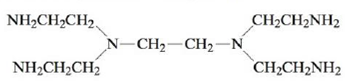# Chelating ligands often form more stable complex ions than the corresponding monodentate ligands with the same donor atoms. For example, Ni 2 + ( a q ) + 6 NH 3 ( a q ) ⇌ Ni ( NH 3 ) 6 2 + ( a q ) K = 3.2 × 10 8 Ni 2 + ( a q ) + 3 en ( a q ) ⇌ Ni ( e n ) 3 2 + ( a q ) K = 1.6 × 10 18 Ni 2 + ( a q ) + penten ( a q ) ⇌ Ni ( penten ) 2 + ( a q ) K = 2.0 × 10 19 where en is ethylenediamine and penten is This increased stability is called the chelate effect. Based on bond energies, would you expect the enthalpy changes for the above reactions to be very different? What is the order (from least favorable to most favorable) of the entropy changes for the above reactions? How do the values of the formation constants correlate with ∆S°? How can this be used to explain the chelate effect?### Chemistry: An Atoms First Approach

2nd Edition
Steven S. Zumdahl + 1 other
Publisher: Cengage Learning
ISBN: 9781305079243

#### Solutions

Chapter
Section### Chemistry: An Atoms First Approach

2nd Edition
Steven S. Zumdahl + 1 other
Publisher: Cengage Learning
ISBN: 9781305079243
Chapter 20, Problem 93CP
Textbook Problem
1 views

## Chelating ligands often form more stable complex ions than the corresponding monodentate ligands with the same donor atoms. For example, Ni 2 + ( a q ) + 6 NH 3 ( a q ) ⇌ Ni ( NH 3 ) 6 2 + ( a q ) K = 3.2 × 10 8 Ni 2 + ( a q ) + 3 en ( a q ) ⇌ Ni ( e n ) 3 2 + ( a q ) K = 1.6 × 10 18 Ni 2 + ( a q ) + penten ( a q ) ⇌ Ni ( penten ) 2 + ( a q ) K = 2.0 × 10 19 where en is ethylenediamine and penten isThis increased stability is called the chelate effect. Based on bond energies, would you expect the enthalpy changes for the above reactions to be very different? What is the order (from least favorable to most favorable) of the entropy changes for the above reactions? How do the values of the formation constants correlate with ∆S°? How can this be used to explain the chelate effect?

Interpretation Introduction

Interpretation: Reactions corresponding to the formation of chelating ligands are given.

Based on bond energies, weather the given reactions will have different enthalpy changes or not. Order of the entropy changes for the given reactions is to be stated. The relationship between ΔSο and values of formation constants and its usage to explain chelate effect is to be stated.

Concept introduction: The electrons in the d orbital of a transition metal split into high and low energy orbitals when ligands are attached to it. The energy difference between these two levels depends upon the properties of both metal and the ligands. If the ligand is strong, then splitting will be high and the complex will be low spin. If the ligand is weak, then splitting will be less and the complex will be high spin.

To determine: If the given reactions will have different enthalpy changes or not.

### Explanation of Solution

Explanation

The change in enthalphy depends upon the nature of ligand, nature of metal and size of ligand. There is difference in the size of ligands amine, ethylenediammine and penten, therefore there enthalphy changes of the given reactions are different.

The order of the entropy changes for the given reactions is Ni(NH3)62+(aq)<Ni(en)32+(aq)<Ni(penten)2+(aq) .

The change in entropy is directly proportional to the change in the number of molecules in the reactants and in the product.

For the reaction,

Ni(H2O)6+2(aq)+6NH3(aq)Ni(NH3)62+(aq)+6H2O

The number of molecules in the reactant is 1+6=7 . The number of molecules in products is 1+6=7 . There is no difference in number of molecules.

For the reaction,

Ni(H2O)6+2(aq)+3enNi(en)32+(aq)+6H2O

The number of molecules in the reactant is 1+3=4

### Still sussing out bartleby?

Check out a sample textbook solution.

See a sample solution

#### The Solution to Your Study Problems

Bartleby provides explanations to thousands of textbook problems written by our experts, many with advanced degrees!

Get Started

Find more solutions based on key concepts
What behaviors would be most helpful in preventing osteoporosis?

Understanding Nutrition (MindTap Course List)

Which of these mineral supplements can easily cause accidental poisoning in children? a. iron b. sodium c. magn...

Nutrition: Concepts and Controversies - Standalone book (MindTap Course List)

Write the correct IUPAC name for each of the following: a. c. b. d.

Chemistry for Today: General, Organic, and Biochemistry

Describe how bone first develops.

Human Biology (MindTap Course List)

(a) If a hole could be dug to the center of the Earth, would the force on an object of mass m still obey Equati...

Physics for Scientists and Engineers, Technology Update (No access codes included)

How has the advent of containerized shipping changed world economics?

Oceanography: An Invitation To Marine Science, Loose-leaf Versin

Draw the vector A=16.5i33.0j on a coordinate system.

Physics for Scientists and Engineers: Foundations and Connections